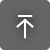# TL Catenary 3D

Todor Latev

Operační systém:
Jazyk:

## Popis

From Wikipedia:

In physics and geometry, a catenary is the curve that an idealized hanging chain or cable assumes under its own weight when supported only at its ends. The catenary curve has a U-like shape, superficially similar in appearance to a parabolic arch, but it is not a parabola. Mathematically, the catenary curve is the graph of the hyperbolic cosine function. Catenaries and related curves are used in architecture and engineering, in the design of bridges and arches, so that forces do not result in bending moments.
In the Catenary equation the parameter a represents the length of chain whose weight on Earth is equal in magnitude to the tension/compression at the Sag/Crest point.

This app will compute and draw the catenary curve as a polyline in Autodesk® AutoCAD® by numerically solving the transcendental equation using a variation of the dampened Newton-Raphson root-finding algorithm. The app supports both "hanging" (positive parameter along the direction of the gravity vector) and "standing" (negative parameter along the direction of the gravity vector) curves.

All 2D Catenary commands assume a positive direction of gravity along the vector (0,-1) of the current/active UCS and operate within the current UCS XY plane
All 3D Catenary commands assume a positive direction of gravity along the vector (0,0,-1) of the current/active UCS and operate within the current UCS 3D space

The following different modes of defining the catenary are supported:

1. One point and parameter a
2. Two points and curve length
3. Two points and a slope of the curve tangent at a specified location
4. Two points and curve sag
5. Two points and curve bulge
6. Two points and curve vertical bulge
7. Two points and curve area
8. Three points
9. Best Fit

Please refer to the Catenary diagram for definitions of all parameters

Each method is implemented as a separate command. All modes/commands are fully interactive, and the user is able to dynamically preview the result on screen. Upon completion of the command a technical report is generated with all input parameters used as well as the final mathematical equation of the computed catenary curve and the computed values of all catenary elements. Note that values of the computed elements such as length, area, bulge etc may differ slightly from the linework generated in AutoCAD due to the numerical approximation of the final polyline geometry. In those cases the computed values should be considered more accurate. Increasing the number of vertices of the catenary polyline should also help minimize these deviations. The number of vertices of the generated polyline is adjustable within a 5-1000 points range. All numerical data is reported using the current AutoCAD setting for the linear precision as specified in the "units" dialog box.

Features:

• Scriptable command line interface
• Interactive commands with dynamic on-screen preview of the result
• All commands operate in the current/active AutoCAD UCS
• Full support for both "hanging" and "standing" catenary curves
• Multiple CPUs/Multi-core CPU support
• Technical report for each successfully computed catenary curve
• Rigorous least squares data-fitting analysis for unlimited number of catenary data points
• Old values of the various input parameters are retained and can be reused by accepting the defaults at the command prompt
Přečíst si dokument nápovědy

## Informace o této verzi

Verze 2019.9.10.1, 24.03.2020